## The Photon Field in the Quantum Hamiltonian

The Hamiltonian for a charged particle in an ElectroMagnetic field is given byLets assume that there is some ElectroMagnetic field around the atom. The field is not extremely strong so that theterm can be neglected (for our purposes) and we will work in the Coulomb gauge for which. The Hamiltonian then becomesNow we have a potentially time dependent perturbation that may drive transitions between the atomic states.Lets also assume that the field has some frequencyand corresponding wave vector. (In fact, and arbitrary field would be a linear combination of many frequencies, directions of propagation, and polarizations.)whereis a real vector and we have again introduced the factor of 2 for convenience of splitting the cosine into two exponentials.

We need to quantize the EM field into photons satisfying Plank's original hypothesis,. Lets start by writingin terms of the number of photons in the field (at frequencyand wave vector). Using classical E&M to compute the energy in a field represented by a vector potential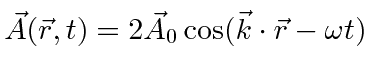, we find that the energy inside a volume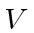isWe may then turn this around and writein terms of the number of photons.We have introduced the unit vector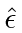to give the direction (or polarization) of the vector potential. We now have a perturbation that may induce radiative transitions. There are terms with both negative and positiveso that we expect to see both stimulated emission of quanta and absorption of quanta in the the presence of a time dependent EM field.

But what about decays of atoms with no applied field? Here we need to go beyond our classical E&M calculation and quantize the field. Since the terms in the perturbation above emit or absorb a photon, and the photon has energy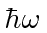, lets assume the number of photons in the field is theof a harmonic oscillator. It has the right steps in energy. Essentially, we are postulating that the vacuum contains an infinite number of harmonic oscillators, one for each wave vector (or frequency...) of light.

We now want to go from a classical harmonic oscillator to a quantum oscillator, in which the ground state energy is not zero, and the hence the perturbing field is never really zero. We do this by changing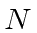to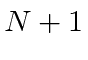in the term that creates a photon in analogy to the raising operatorin the HO. With this change, our perturbation becomes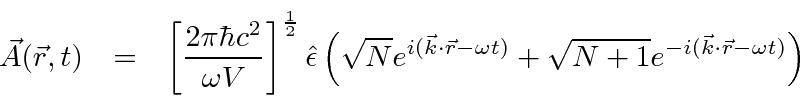Remember that one exponential corresponds to the emission of a photon and the other corresponds to the the absorption of a photon. We viewas an operator which either creates or absorbs a photon, raising or lowering the harmonic oscillator in the vacuum.

Now there is a perturbation even with no applied field ().We can plug this right into our expression for the decay rate (removing theinto the delta function as was done when we considered a general sinusoidal time dependent perturbation). Of course we have this for all frequencies, not just the one we have been assuming without justification. Also note that our perturbation still depends on the volume we assume. This factor will be canceled when we correctly compute the density of final states.

We have taken a step toward quantization of the EM field, at least when we emit or absorb a photon. With this step, we can correctly compute the EM transition rates in atoms. Note that we have postulated that the vacuum has an infinite number of oscillators corresponding to the different possible modes of EM waves. When we quantize these oscillators, the vacuum has a ground state energy density in the EM field (equivalent to half a photon of each type). That vacuum EM field is then responsible for the spontaneous decay of excited states of atoms through the emission of a photon. We have not yet written the quantum equations that the EM field must satisfy, although they are closely related to Maxwell's equations.

Jim Branson 2013-04-22